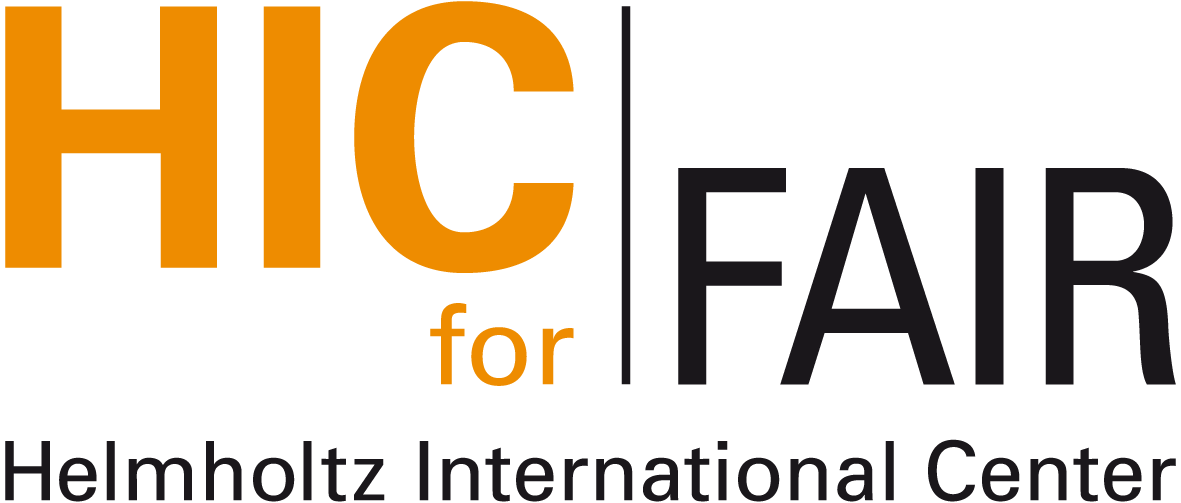$\newcommand{\dd}{\mathrm{d}}$ $\DeclareMathOperator{\sign}{sign}$

#Nuclear Physics Colloquium and Transport Meeting

Venue: Otto-Stern-Zentrum OSZ S1
Time: Wednesday, June 03, 11:00 (s.t.) (plus 10min for discussion)
Contact:

# QCD equation of state and cosmological parameters in early Universe

## Paolo Castorina (Università di Catania, Italy)

After discussing the effective degrees of freedom in the deconfined phase, the time evolution of cosmological parameters in early Universe  is studied, near and above the critical temperature, by an equation of state (EoS) which takes into account the finite baryon density and the background magnetic field.

The non-perturbative dynamics is described by the Field Correlator Method (FCM) which gives, with a small number of free parameters, a good fit of lattice data. The entire system has two components, i.e. the quark-gluon plasma and the electroweak sector, and the solutions of the Friedmann equation show that the scale factor, $a(t)$, and $H(t) = (1/a) \dd a/\dd t$ are weakly dependent on the EoS, but the deceleration parameter, $q(t)$, and the jerk, $j(t)$, are strongly modified above the critical temperature $T_c$, corresponding to a critical time $t_c \simeq 20$-$25 \; \mu \mathrm{s}$.

The time evolution of the cosmological parameters suggests that above and around $T_c$ there is a transient state of acceleration typical of a matter dominated Universe; this is entailed by the QCD strong interaction driven by the presence of massive colored objects.

Nuclear Physics Colloquium Homepage